# Python Arithmetic Operators

Operators are one of the most important aspect of programming language. The operators give the ability to do various types of computation in programming languages.

The arithmetic operator is the most common and basic type of operators you will come across in programming languages. Python also supports the basic arithmetic operators and some new operators.

Here is the list of common arithmetic operators in python.

Special arithmetic operators in python.

Now we will see some examples of each other and discuss the properties.

The addition operator will add two numbers and display the result of the expression.

num1 = 20
num2 = 23
result = num1 + num2
print(result)

The output of the program is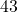. See below.

============== RESTART: C:/Python_projects/Addition_operator.py ==============
43
>>> 

The python will add floating point number and integer and result will be float. This is because the floating point representation always take more memory bits than integers.

num3 = 345
num4 = 67.255
result2 = num3 + num4
print("The result is",result2)

The output of the above program is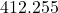. See output below.

=========== RESTART: C:/Python_projects/addition_float_integer.py ===========
412.255
>>> 

The addition operator is also good for strings. It can be used to concatenate two strings. For example.

str1 = "Demo "
str2 = "Program"
result3 = str1 + str2
print("This is a",result3)

The above code will add two strings into a common string and display the output.

=============== RESTART: C:/Python_projects/Add_Two_Strings.py ===============
This is a Demo Program
>>> 

#### Subtraction Operator (-)

The subtraction operator also known as minus. It will subtract from first variable, a quantity equal to the second variable.

num5 = 200
num6 = 50
result4 = num5 - num6
print("The final value is",result4)

A quantity of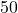which is num6 will be subtracted from num5 and output is displayed. See the output below.

============ RESTART: C:/Python_projects/subtraction_operator.py ============
The final value is 150
>>> 

#### Multiplication Operator (*)

The multiplication operator will multiply a number with another number to get a final value.

num7 = 10
num8 = 22
result5 = num7 * num8
print("The product is", result5)

The first number is multiplied with the second number to produce the product. Then the program output the result.

=========== RESTART: C:/Python_projects/Multiplication_operator.py ===========
The product is 220
>>> 

#### Division Operator(/)

The output of division operator is always floating point number. The operator divides first number with the second number and return the quotient value.

For example.

dividend = 2300
divisor = 100
result6 = dividend/divisor
print("The quotient is", result6)

The output is in floating point as follows.

============== RESTART: C:/Python_projects/Division_Operator.py ==============
The quotient is 23.0
>>> 

#### Modulus Operator (%)

The modulus operator will give remainder of a division. It is useful in some mathematical expression where we need to check the class of number( just by checking the number we can decide the class).

For example.

number1 = 23
number2 = 5
remainder_value = number1 % number2
print("The remainder is", remainder_value)

The output of the above is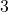which is remainder of the division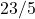. See the output below.

============= RESTART: C:/Python_projects/Remainder_Operator.py =============
The remainder is 3
>>> 

### Special Operators

The two special operators are floor and the exponential. In many programming languages like C/C++ the exponential and floor are part of the math library. However, despite having a math module, python created these two operators due to the popularity and usage of these operator in programming world.

#### Floor Operator (//)

Since, the division of python is floating point number, sometimes you need an integer output. The floor operator will result the lowest possible integer value of a division. Take for example,which will return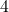as the result, instead of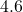.

number_1 = 23
number_2 = 5
quotient = 23/5
result_floored = 23 // 5
print(quotient)
print(result_floored)

The output of the above is given below. Note the difference between quotient and the floor.

============== RESTART: C:/Python_projects/Floored_operator.py ==============
4.6
4
>>> 

#### Exponential (**)

Exponential are frequently used in the both mathematics and computer programming. Exponents have two values – integer part and power part. An integer is raised to power of another number. For example,

23  \hspace{3px} means \hspace{3px} 2 * 2 * 2 = 8
number = 2
power = 4
expo = number ** power
print("The output is", expo)

The output is equal to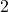raised to power ofwhich is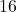. See answer below.

================= RESTART: C:\Python_projects\Exponetial.py =================
The output is 16
>>> 

### Arithmetic Operator With Mixed Data Types

Now, you are familiar with the data types and arithmetic operators. The arithmetic operators are naturally for numbers. However, you can also use them with certain combination of other data types.

Number With String

out1 = "Jet Lee" * 5
print(out1)

The above code will not give error but print following.

====== RESTART: C:\Python_projects\Number_with_String_multiplication.py ======
Jet LeeJet LeeJet LeeJet LeeJet Lee
>>> 

Number With Boolean

You can even multiply with a Boolean value and number because Boolean isor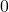when converted to numbers. Therefore,

out2 = True * 5
print(out2)

The output will be as follows.

============= RESTART: C:/Python_projects/Number_with_Boolean.py =============
5
>>> 

You may try different combination and will find that the only combination that does not work is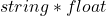.

out3 = "Hello" * 3.3
print(out3)

The output is not printed, but you will get an error.

 RESTART: D:/Users/username/AppData/Local/Programs/Python/Python37-32/string_with_float_multiplicaition.py
Traceback (most recent call last):
File "D:/Users/username/AppData/Local/Programs/Python/Python37-32/string_with_float_multiplicaition.py", line 1, in <module>
out3 = "Hello" * 3.3
TypeError: can't multiply sequence by non-int of type 'float'
>>> 

In the next few articles, we will talk more about relational operators and Boolean operators in detail.